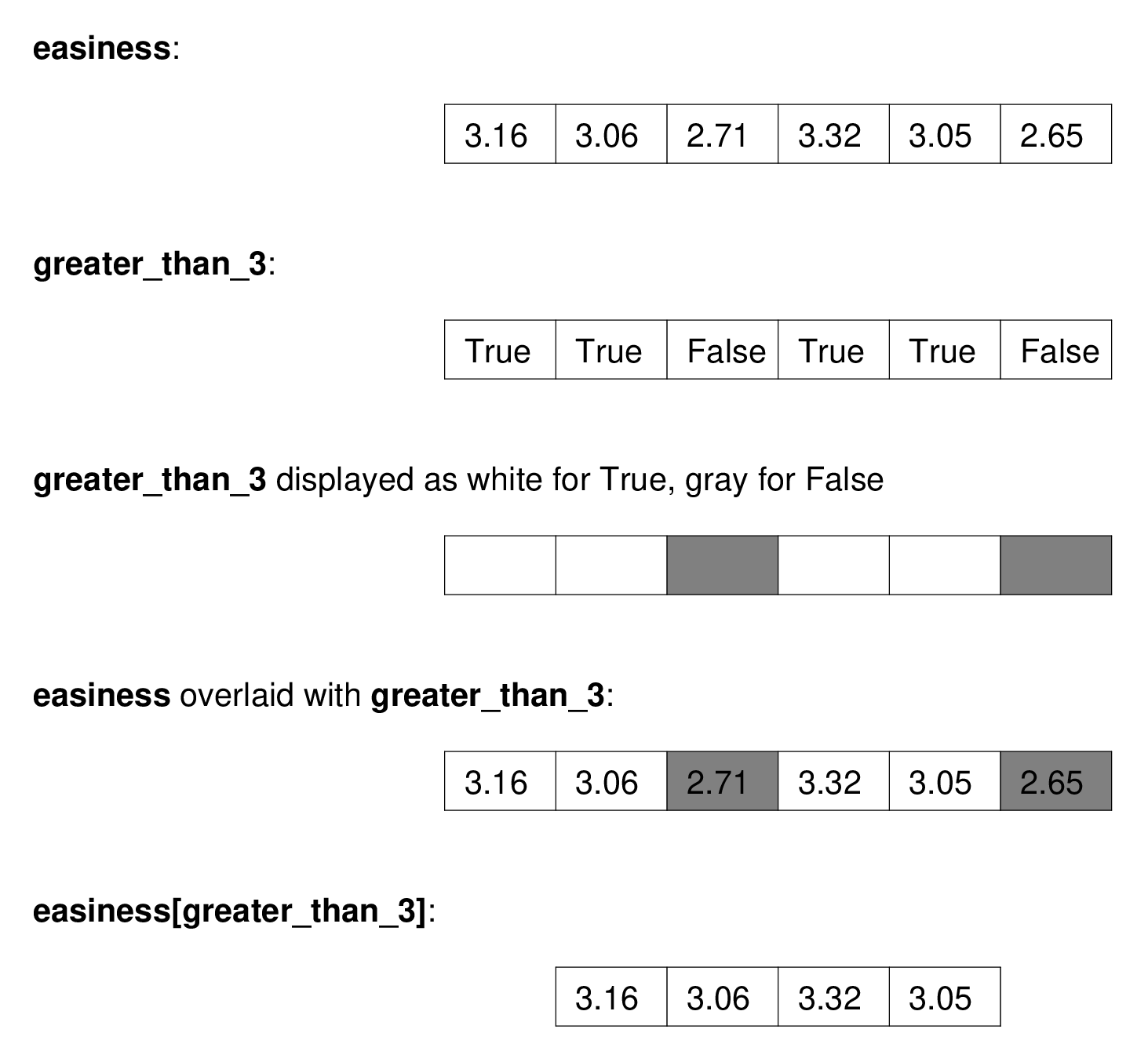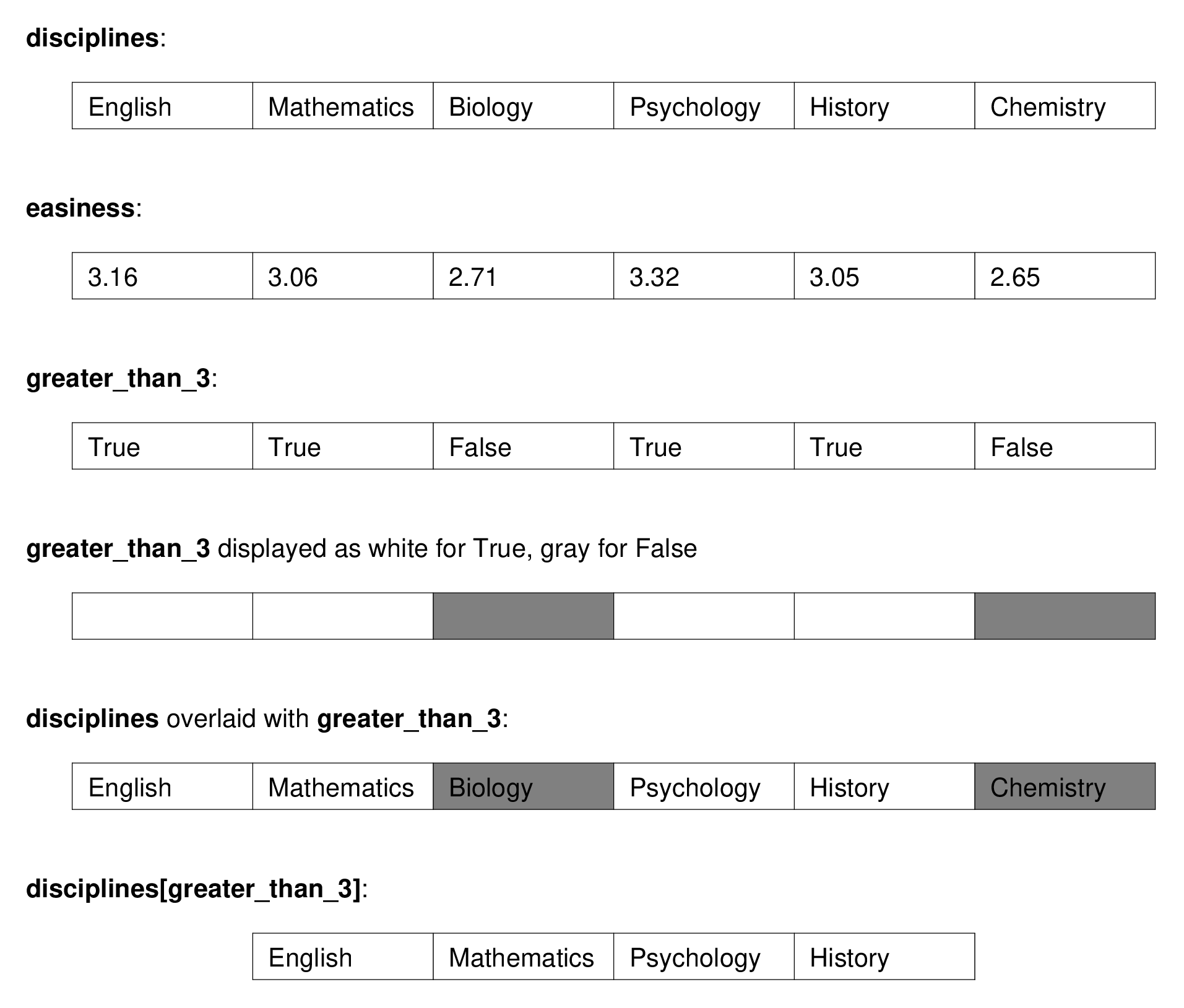# Indexing with Boolean arrays#

As usual with arrays, we need the Numpy library:

import numpy as np


Remember Boolean values, and Comparison operators from Brisk introduction to Python. We will be using these values and operators, in and with arrays.

## Select values with Boolean arrays#

Here we are using Boolean arrays to index into other arrays. You will see what we mean by that by the end of this section.

We often want to select several elements from an array according to some criterion.

The most common way to do this, is to do array slicing, using a Boolean array between the square brackets.

It can be easier to understand this by example than by description.

We are going to use some example data from student ratings of their professors.

You can go to the link for the long story, but the short story is that the dataset is a table where the rows are academic disciplines, and the columns contain the average student rating values for the corresponding discipline.

Here we have extracted the ratings for the six largest subjects — the subjects with the largest number of rated professors.

This is the array of discipline names for those six largest subjects:

disciplines = np.array(
['English', 'Mathematics', 'Biology',
'Psychology', 'History', 'Chemistry'])
disciplines

array(['English', 'Mathematics', 'Biology', 'Psychology', 'History',
'Chemistry'], dtype='<U11')


One of the ratings the students gave was of how easy the course was, on a five point scale from 1 (hard) to 5 (easy).

These are the average “Easiness” scores for the six largest courses named above:

easiness = np.array([3.16, 3.06, 2.71, 3.32, 3.05, 2.65])


The top (largest) discipline is:

disciplines

'English'


The Easiness rating for that course is:

easiness

3.16


and so on.

## Boolean arrays#

Boolean arrays are arrays that contain values that are one of True or False.

Here is a Boolean array, created from applying a comparison to an array:

greater_than_3 = easiness > 3
greater_than_3

array([ True,  True, False,  True,  True, False])


This has a True value at the positions of elements > 3, and False otherwise.

We can do things like count the number of True values in the Boolean array:

np.count_nonzero(greater_than_3)

4


Now let us say that we wanted to get the elements from easiness that are greater than 3. That is, we want to get the elements in easiness for which the corresponding element in greater_than_3 is True.

We can do this with Boolean array indexing. The Boolean array goes between the square brackets, after the array name. As a reminder:

# The easiness array
easiness

array([3.16, 3.06, 2.71, 3.32, 3.05, 2.65])

# The greater_than_3 Boolean array
greater_than_3

array([ True,  True, False,  True,  True, False])


We put the Boolean array between square brackets, after the array we want to get values from, like this:

# Boolean indexing into the easiness array.
easiness[greater_than_3]

array([3.16, 3.06, 3.32, 3.05])


We have selected the numbers in easiness that are greater than 3.

See the picture below for an illustration of what is happening:We can use this same Boolean array to index into another array. For example, here we show the discipline names corresponding to the courses with Easiness scores greater than 3:

disciplines[greater_than_3]

array(['English', 'Mathematics', 'Psychology', 'History'], dtype='<U11')


See the picture below for an illustration of how this works:## Setting values with Boolean arrays#

You have seen, above, that Boolean indexing can select values from an array:

# Create the Boolean array
another_array = np.array([2, 3, 4, 2, 1, 5, 1, 0, 3])
are_gt_2 = another_array > 2
are_gt_2

array([False,  True,  True, False, False,  True, False, False,  True])

# Get the values by indexing with the Boolean array.
# Return only the values of 'another_array' where the Boolean array has True.
another_array[are_gt_2]

array([3, 4, 5, 3])


Given what you know, what do you think would happen with:

another_array[are_gt_2] = 10
another_array


Try it.